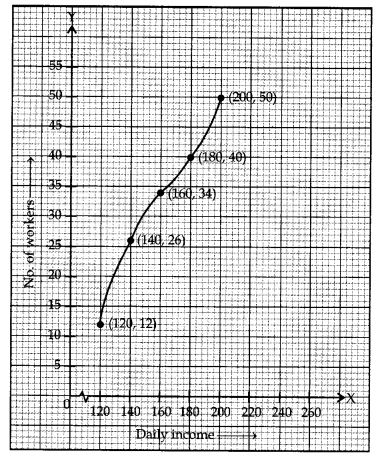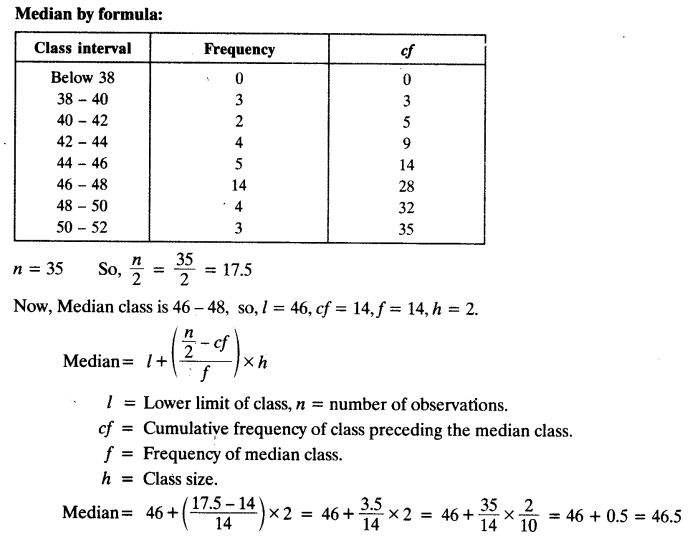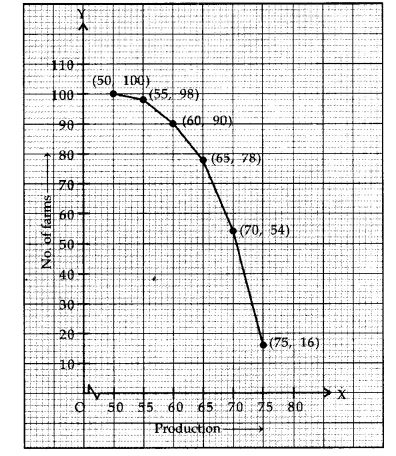# NCERT Solutions For Class 10 Maths Chapter 14 Statistics Ex 14.4

Get Free NCERT Solutions for Class 10 Maths Chapter 14 Ex 14.4 PDF. Statistics Class 10 Maths NCERT Solutions are extremely helpful while doing your homework. Exercise 14.4 Class 10 Maths NCERT Solutions were prepared by Experienced ncert-books.in Teachers. Detailed answers of all the questions in Chapter 14 Maths Class 10 Statistics Exercise 14.4 provided in NCERT TextBook.

Question 1.
The following distribution gives the daily income of 50 workers of a factory.Convert the distribution above to a less than type cumulative frequency distribution, and draw its ogive.
Solution:Question 2.
During the medical check-up of 35 students of a class, their weights were recorded as follows:Draw a less than type ogive for the given data. Hence obtain the median weight from the graph and verify the result by using the formula.
Solution:Question 3.
The following table gives production yield per hectare of wheat of 100 farms of a village.Change the distribution to a more than type distribution, and draw its ogive.
Solution:error: Content is protected !!
+